# Test: Capacitors & Inductors

## 10 Questions MCQ Test Topicwise Question Bank for Electrical Engineering | Test: Capacitors & Inductors

Description
Attempt Test: Capacitors & Inductors | 10 questions in 30 minutes | Mock test for Electrical Engineering (EE) preparation | Free important questions MCQ to study Topicwise Question Bank for Electrical Engineering for Electrical Engineering (EE) Exam | Download free PDF with solutions
QUESTION: 1

### An Inductor works as a ________ circuit for DC supply.

Solution:

Induced voltage across an inductor is zero if the current flowing through it is constant, i.e. Inductor works as a short circuit for DC supply.

QUESTION: 2

### The equivalent circuit of the capacitor shown below is: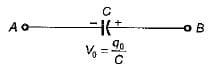Solution:

Due to initial condition, at t = 0 capacitor will act as a constant voltage source (at t = 0, capacitor acts as short-circuit). Hence, option (d) is correct.

QUESTION: 3

### The strength of current in 1 Henry inductor changes at a rate of 1 A/sec. The magnitude of energy stored in the inductor after 3 sec is:

Solution: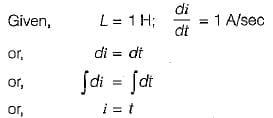∴ Current in the inductor after 3 sec is:

1= 3 A

Hence, energy is stored after 3 sec: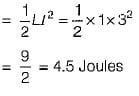QUESTION: 4

The current and voltage profile of an element vs time has been shown in given figure. The element and its value are respectively: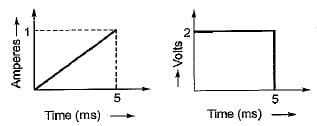Solution:
• Since V is not proportional to R therefore, the element can’t be a resistor.
• At t = 5 ms, even if i ≠ 0, the element behaves as a short circuit therefore, the element can’t be a capacitor (since at t = 0 only capacitor behaves as short circuit).
• The current at t = 0 is zero and at t = 5 ms voltage across the element is zero therefore, the element must be an inductor (at t = 0, an inductor acts as open circuit and at t =∞ it behaves as short circuit).
• From the given voltage and current profile, we have: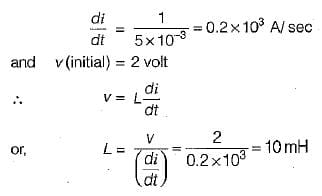QUESTION: 5

Figure shown below exhibits the voltage-time profile of a source to charge a capacitor of 50 μF. The value of charging current in amperes is: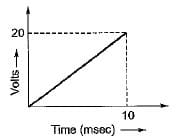Solution:

From given figure: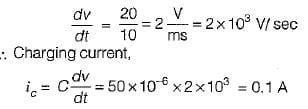QUESTION: 6

The equivalent capacitance across the given terminals A-B is: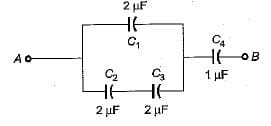Solution:
• The equivalent combination of C2 and C3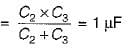• The equivalent combination of this 1 μF and  C1 = 2μF is C1+ 1 μF = 3μF
• Hence, the equivalent capacitance between terminals A and B is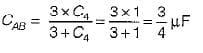QUESTION: 7

The charging time required to charge the equivalent capacitance between the given terminals a-b by a steady direct current of constant magnitude of 10 A is given by: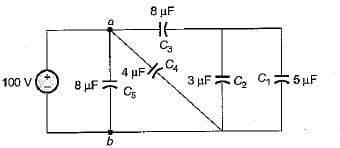Solution:

Equivalent capacitance between terminals a-b is: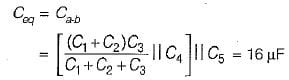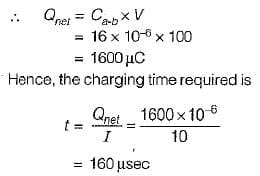QUESTION: 8

An ac voltage of 220 V is applied to a pure inductance of 50 H. If the current is 5 A, the instantaneous value of voltage and current will be respectively given by:

Solution:

Max. value of current: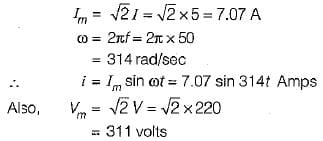Assuming voltage as the reference phasor: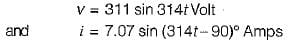QUESTION: 9

The voltage and current through a circuit element is v= 100 sin (314 t + 45°) volts and i = 10 sin (314 t - 45 ° ) amps.
The type of circuit element and its value will be respectively:

Solution: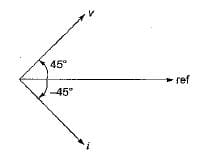The phase difference between v and i is: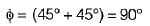Since v leads i therefore, the circuit element is an inductor.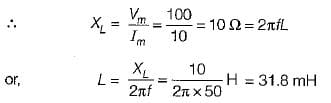QUESTION: 10

The equivalent inductance for the inductive circuit shown below at terminal “ 1 - 2 ” is: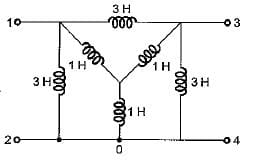Solution:

Converting the internal star connected inductance to an equivalent delta, the circuit reduces as shown below.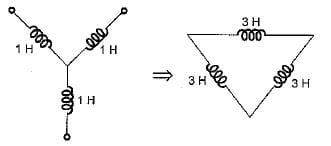Hence, equivalent circuit becomes as shown below.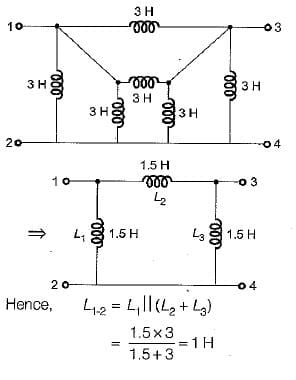Use Code STAYHOME200 and get INR 200 additional OFF Use Coupon Code